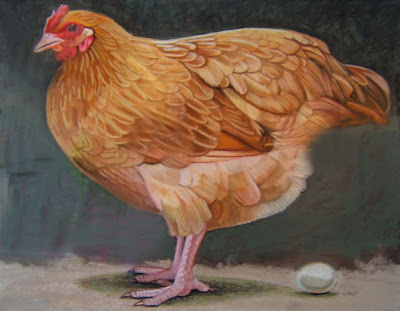## tisdag 28 juni 2011

### The Hen and the Egg of GravitationAs summer holidays are coming up and string theorists gather in Uppsala, it may be time to reflect in the hammock about the origin of gravitation and then recall the knol The Hen and Egg of Gravitation reporting on the following idea exposed in Many-Minds Relativity:

The gravitational potential P is related to distribution of mass M by the differential equation
• - Laplace P = M
where Laplace is the Laplace operator. This equation can be read in two ways:
1. Given M, the potential P is obtained by solving the differential equation - Laplace P = M.
2. Given P, the mass distribution M is obtained by applying - Laplace to P.
Here 1 is a non-local operation (solving a differential equation) reflecting action at distance, while 2 is a local operation (differentiation) without action at distance.

While in the hammock the reader is invited to reflect about 1 vs 2: Which comes first, the potential (the hen) or the mass (the egg)?